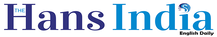Top

# Understanding moving averagesHighlights

## All of us are familiar with the term average since our school days Moving averages in stock market is an extension of it Moving averages are trend indicators They are extensively used in technical analysis by traders and investors This trend indicator is simple and effective

All of us are familiar with the term average since our school days. Moving averages in stock market is an extension of it. Moving averages are trend indicators. They are extensively used in technical analysis by traders and investors. This trend indicator is simple and effective.

Let us understand this term in detail with the help of an example. The below table shows the closing price of Indian Oil Corporation (IOC) during the last five trading sessions.

In the above example, the average price for five days is 663.25/ 5 = 132.05 As 8th and 9th were Saturday and Sunday, the next trading session would be on Monday that is on December 10, 2018. So, now we move forward and have a new data point from December 10 onwards.

This implies that the new latest five days would be December 10, 11, 12, 13 and 14, 2018. In the above example, the average price is calculated as follows; 681.05/ 5 = 136.21

The data belonging to December 3 – 7, will be dropped as objective is to calculate the latest 5- day average. So now the average price of IOC for the last 5 trading sessions is 136.21. So, here we observe to include the latest data ranging from December 10 to 14 and discard the data from December 3 - 7 to calculate the five-day average.

As we are moving to the latest data and discarding the oldest to calculate the latest 5-day average, leading the name -- moving average to be coined.

In the above example, moving average has been calculated by considering the closing price. Sometimes other parameters like high, low and open are also considered. Generally, the closing price is considered because it indicates the final price of the stock at closing time.

Whenever there is a change in the closing price, the moving average changes. The averages for the five day (or it could be anything like 5, 10, 50 days) are then joined to form a smooth curving line known as a moving average line and it continues to move as the time progresses. (The author is a homemaker who dabbles in stock market investments in free time)